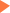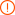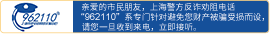# SAT数学练习题 含详细答案解析(1)

2017年08月09日14:59 来源：互联网## SAT/ACT备考资料免费领取 请选择资料！

1. If f(x) = x² – 3, where x is an integer, which of the following could be a value of f(x)?

I 6

II 0

III -6

A. I only

B. I and II only

C. II and III only

D. I and III only

E. I, II and III

Choice I is correct because f(x) = 6 when x=3. Choice II is incorrect because to make f(x) = 0, x² would have to be 3. But 3 is not the square of an integer. Choice III is incorrect because to make f(x) = 0, x² would have to be –3 but squares cannot be negative. (The minimum value for x2 is zero; hence, the minimum value for f(x) = -3)

2. For how many integer values of n will the value of the expression 4n + 7 be an integer greater than 1 and less than 200?

A. 48

B. 49

C. 50

D. 51

E. 52

1 < 4n + 7 < 200. n can be 0, or -1. n cannot be -2 or any other negative integer or the expression 4n + 7 will be less than1. The largest value for n will be an integer < (200 - 7) /4. 193/4 = 48.25, hence 48. The number of integers between -1 and 48 inclusive is 50

3. In the following correctly worked addition sum, A,B,C and D represent different digits, and all the digits in the sum are different. What is the sum of A,B,C and D?

A. 23

B. 22

C. 18

D. 16

E. 14

First you must realize that the sum of two 2-digit numbers cannot be more that 198 (99 + 99). Therefore in the given problem D must be 1. Now use trial and error to satisfy the sum 5A + BC = 143. A + C must give 3 in the units place, but neither can be 1 since all the digits have to be different. Therefore A + C = 13. With one to carry over into the tens column, 1 + 5 + B = 14, and B = 8. A + C + B + D = 13 + 8 + 1 = 22

4. 12 litres of water a poured into an aquarium of dimensions 50cm length , 30cm breadth, and 40 cm height. How high (in cm) will the water rise?

(1 litre = 1000cm³)

A. 6

B. 8

C. 10

D. 20

E. 40

Total volume of water = 12 liters = 12 x 1000 cm3. The base of the aquarium is 50 x 30 = 1500cm3. Base of tank x height of water = volume of water. 1500 x height = 12000; height = 12000 / 1500 = 8

5. Six years ago Anita was P times as old as Ben was. If Anita is now 17 years old, how old is Ben now in terms of P ?

A. 11/P + 6

B. P/11 +6

C. 17 - P/6

D. 17/P

E. 11.5P

Let Ben’s age now be B. Anita’s age now is A. (A - 6) = P(B - 6)

But A is 17 and therefore 11 = P(B - 6). 11/P = B-6

(11/P) + 6 = B

### 相关文章未输入验证码未输入验证码未输入验证码

## SAT30天热搜沪公网安备 31010602002658号